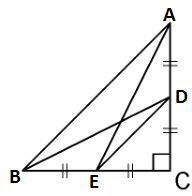# D and E are points on the sides CA and CB respectively of a triangle ABC right angled at C. Prove that AE2 + BD2 = AB2 + DE2.

To prove: (AE)+ (BD)= (AB)+ (DE)2

Construction: Join AE, BD and DEProof:

In ∆ACE, by Pythagoras theorem

(AE)= (AC)+ (EC)2 -(1)

In ∆DCB, by Pythagoras theorem

(BD)= (DC)+ (BC)2 -(2)

In ∆ACB, by Pythagoras theorem

(AB)= (AC)+ (CB)2 -(3)

In ∆DCE, by Pythagoras theorem

(ED)= (DC)+ (CE)2

(AE)+ (BD)= (AC)+ (EC)+ (DC)+ (DC)2

= (AC+ BC2) + (EC+ DC2)

= AB+ DE2 -(from 3 and 4)